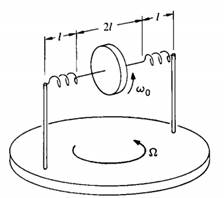### Create an Account

Home / Questions / A flywheel of moment of inertia l0 rotates with angular velocity ω0 at the middle of an ax...

# A flywheel of moment of inertia l0 rotates with angular velocity ω0 at the middle of an axle of length 2 Each end of the axle is attached to a support by a spring which is stretched to length

A flywheel of moment of inertia l0 rotates with angular velocity ω0 at the middle of an axle of length 2l. Each end of the axle is attached to a support by a spring which is stretched to length I and provides tension T. You may assume that T remains constant for small displacements of the axle. The supports are fixed to a table which rotates at constant angular velocity, Ω, where Ω≪ω0. The center of mass of the flywheel is directly over the center of rotation of the table. Neglect gravity and assume that the motion is completely uniform so that nutational effects are absent. The problem is to find the direction of the axle with respect to a straight line between the supports.May 19 2020 View more View LessSubscribe To Get Solution